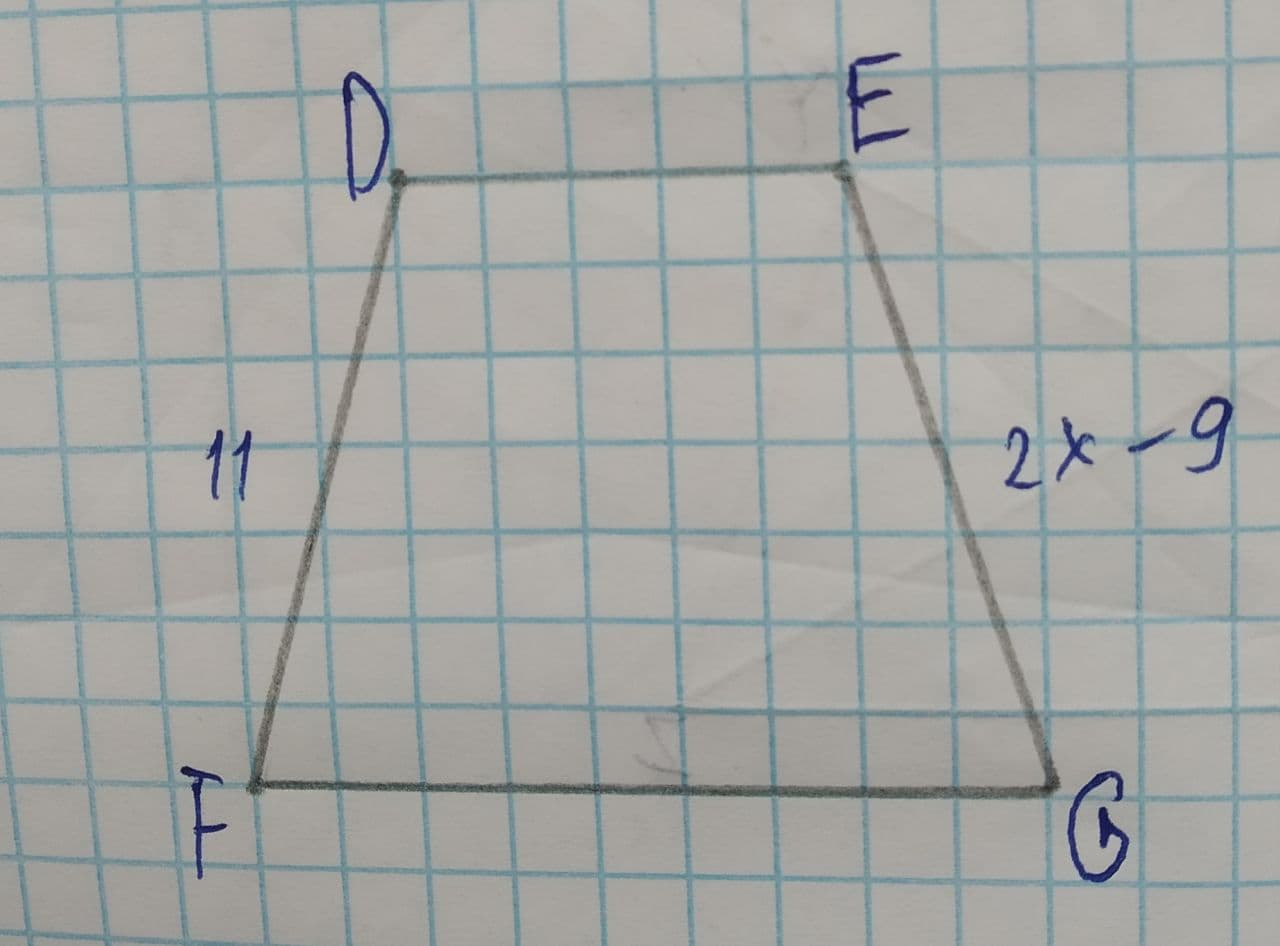# Prove the congruence \overline{DF}\cong\overline{EG}nitraiddQ 2021-07-18 Answered
To Proof: If $$\displaystyle\overline{{{D}{F}}}\stackrel{\sim}{=}\overline{{{E}{G}}}$$ then x = 10
Given:Given: $$\displaystyle\overline{{{D}{F}}}\stackrel{\sim}{=}\overline{{{E}{G}}}$$

• Questions are typically answered in as fast as 30 minutes

### Plainmath recommends

• Get a detailed answer even on the hardest topics.
• Ask an expert for a step-by-step guidance to learn to do it yourself.Ayesha Gomez
Since $$\displaystyle\overline{{{D}{F}}}\stackrel{\sim}{=}\overline{{{E}{G}}}$$
Therefore by using the defination of congruence.
DF=EG
Thus
11=2x-9
11+9=2x
2x=20
x=10
Hence proved.